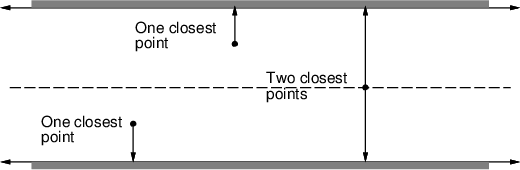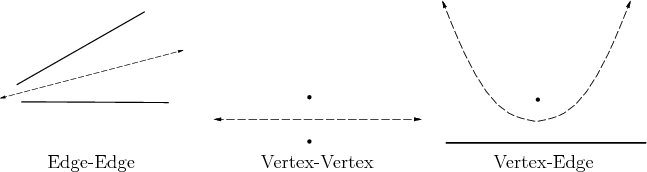A maximum-clearance roadmap tries to keep as far as possible from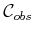, as shown for the corridor in Figure 6.8. The resulting solution paths are sometimes preferred in mobile robotics applications because it is difficult to measure and control the precise position of a mobile robot. Traveling along the maximum-clearance roadmap reduces the chances of collisions due to these uncertainties. Other names for this roadmap are generalized Voronoi diagram and retraction method . It is considered as a generalization of the Voronoi diagram (recall from Section 5.2.2) from the case of points to the case of polygons. Each point along a roadmap edge is equidistant from two points on the boundary of. Each roadmap vertex corresponds to the intersection of two or more roadmap edges and is therefore equidistant from three or more points along the boundary of.

The retraction term comes from topology and provides a nice intuition about the method. A subspace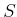is a deformation retract of a topological spaceif the following continuous homotopy,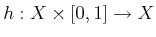, can be defined as follows :

1.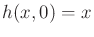for all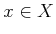.
2.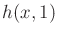is a continuous function that maps every element ofto some element of.
3. For all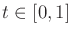,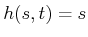for any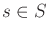.
The intuition is thatis gradually thinned through the homotopy process, until a skeleton,, is obtained. An approximation to this shrinking process can be imagined by shaving off a thin layer around the whole boundary of. If this is repeated iteratively, the maximum-clearance roadmap is the only part that remains (assuming that the shaving always stops when thin slivers'' are obtained).

To construct the maximum-clearance roadmap, the concept of features from Section 5.3.3 is used again. Let the feature set refer to the set of all edges and vertices of. Candidate paths for the roadmap are produced by every pair of features. This leads to a naive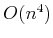time algorithm as follows. For every edge-edge feature pair, generate a line as shown in Figure 6.9a. For every vertex-vertex pair, generate a line as shown in Figure 6.9b. The maximum-clearance path between a point and a line is a parabola. Thus, for every edge-point pair, generate a parabolic curve as shown in Figure 6.9c. The portions of the paths that actually lie on the maximum-clearance roadmap are determined by intersecting the curves. Several algorithms exist that provide better asymptotic running times [616,626], but they are considerably more difficult to implement. The best-known algorithm runs in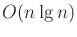time in whichis the number of roadmap curves .

Steven M LaValle 2020-08-14